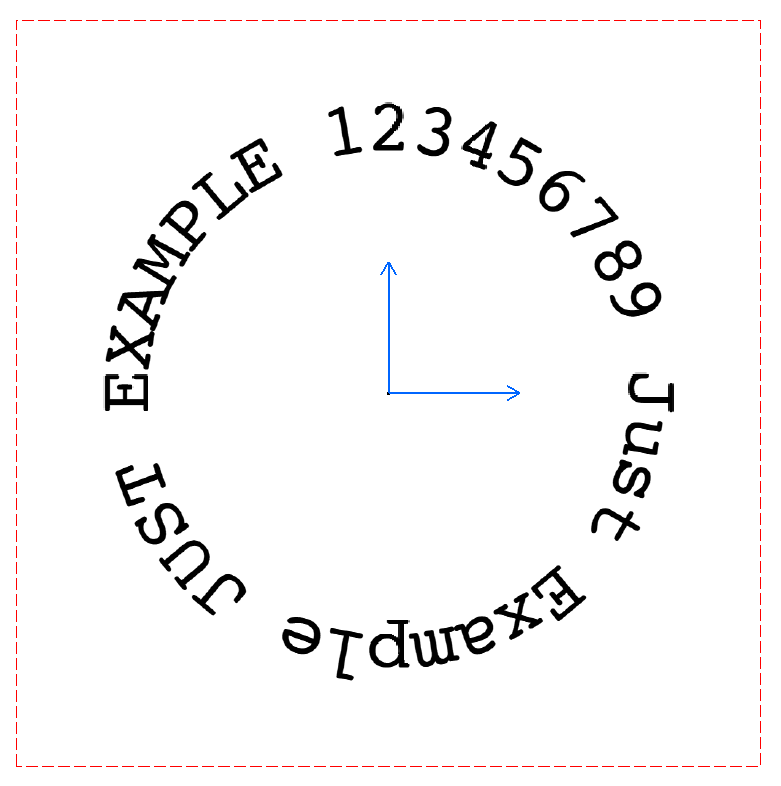# Circular text in CATIA V5 drawings

Circular text in a drawing cannot be done easily using standard CATIA V5 commands. You can maybe put a letter into the required position, use a Rotate command to copy it along a circle and then edit letter text one by one. Or you can utilize the power of VBA.

A code below creates a circular text in a matter of milliseconds. All you have to do is to set some initial properties and let your computer do his job.

## How it works

In the Main procedure, we define variables we need for DrawCircularText routine. A must for all geometric calculations is a constant PI. The PI, unfortunately, does not exist in VBA and we have to specify it ourselves. I have found a nice little trick on how to define it without assigning the PI value directly. A formula pi = 4 * Atn(1) does it.

Other variables like r, spacing, and txt represent properties of circular text:

• r is an inner radius of circular text
• spacing is a central angle of an arc between letters in radians
• txt is our circular text

You can change these variables as you need.

The last row in the Main sub is a call to a DrawCircularText routine, which takes five parameters. Last two of them are declared as Optional and we can omit them in a procedure call if we are happy with predefined font name and font size. And we are :).

``````
Option Explicit

' PI definition on module level
Dim pi As Double

Sub Main()
' set PI variable
pi = 4 * Atn(1)

' get active view
Dim view As DrawingView
Set view = CATIA.ActiveDocument.Sheets.ActiveSheet.Views.ActiveView

Dim r As Double
Dim spacing As Double
Dim txt As String

' set circular text properties, change it as you need!
r = 50
spacing = 10 * (pi / 180)
txt = "Just Example JUST EXAMPLE 123456789"

DrawCircularText view, r, spacing, txt
'    DrawCircularText view, r, spacing, txt, "Algerian (TrueType)", 8
End Sub
``````

The procedure DrawCircularText takes care of all hard work. It draws each letter from input string to the correct location and set its properties like the angle, anchor point, font name, and font size.

Probably the most important here is a parametric equation of a circle which is defined as:

• x = r cos(t)
• y = r sin(t)

where x, y are the coordinates of any point on the circle, r is the radius of the circle and t is the parameter - the angle.

``````
Sub DrawCircularText(view As DrawingView, r As Double, spacing As Double, txt As String, _
Optional fntName As String = "Courier10 BT", Optional fntSize As Long = 10)
' get drawing text collection
Dim drwTexts As DrawingTexts
Set drwTexts = view.Texts

Dim i As Long
Dim letterAngle As Double

For i = 1 To Len(txt)
Dim posX As Double
Dim posY As Double

posX = r * Cos(letterAngle)
posY = r * Sin(letterAngle)

' create letter
Dim letter As DrawingText
Set letter = drwTexts.Add(Mid(txt, i, 1), posX, -posY)
letter.Angle = 270 - letterAngle * 180 / pi

' get DrawingTextProperties object of a letter
Dim textProp As DrawingTextProperties
Set textProp = letter.TextProperties

' set text properties of a letter
With textProp
' set letter mirror mode, do not let CATIA to perform auto flip of text
.Mirror = catTextNoFlip
.AnchorPoint = catBottomCenter
.FontName = fntName
.FontSize = fntSize

' update text properties
.Update
End With

letterAngle = spacing * i
Next
End Sub
``````

If you copy all routines into a standard module and launch Main procedure, you should get a nice circular text like the one below.Circular text in CATIA V5 Drawing